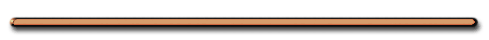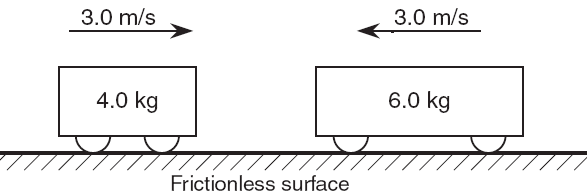II. Conservation of Momentum#22

 Total Momentum Before Interaction =   Total Momentum After Interaction

m1v1 + m2v2 = m1v1′ + m2v2

Momentum is only conserved
in a totally elastic collision

Totally Elastic Collision?What is the momentum of the big fish after it  swallows the little fish?

-4 kgm/s

Total momentum before =
Total momentum after

 Ex 4) What is the magnitude of the total momentum of these carts?Total momentum = m1v1 + m2v2

Total mom. =

(4.0 kg)(3 m/s)+6.0kg(-3.0m/s)

= 12. kgm/s + -18. kgm/s

= -6.0 kgm/s"Left train momentum = 30 kgm/s. What is the momentum  of the right train?"

What's the total momentum before the collision?

After the collision?